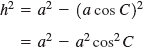### 8.4. Law of Cosines

To use the law of sines we need a known side opposite a known angle. Sometimes we do not have that information, as when, for example, we know three sides and no angle. We can still solve such a triangle using the law of cosines.

#### 8.4.1. Derivation

Consider an oblique triangle ABC as shown in Fig. 8-27. As we did for the law of sines, we start by dividing the triangle into two right triangles by drawing an altitude h to side AC.

In right triangle ABD,

But AD = bCD. Substituting, we getNow, in right triangle BCD, by the definition of the cosine,or

Substituting a cos C for CD in Equation (1) yields

c2 = h2 + (ba cos C)2

Squaring, we have##### Figure 8.27. Derivation of the law of cosines.Let us leave this expression for the moment and write the Pythagorean theorem for the same triangle BCD.

h2 = a2 − (CD)2

Again substituting a cos C for CD, we obtainSubstituting this expression for h2 back into (2), we get

c1 = a2a2cos2C + b2 − 2ab cos ...

Get Technical Mathematics, Sixth Edition now with the O’Reilly learning platform.

O’Reilly members experience live online training, plus books, videos, and digital content from nearly 200 publishers.